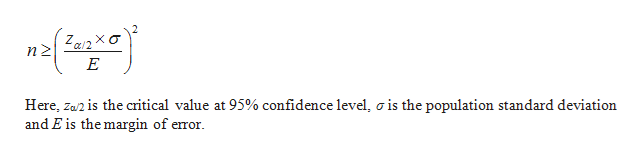# Find the minimum sample size n needed to estimate muμ for the given values of​ c, sigmaσ​, and E. c=0.95​,σ=8.1​, and E=2

Question
50 views

Find the minimum sample size n needed to estimate muμ for the given values of​ c, sigmaσ​, and E. c=0.95​,σ=8.1​, and E=2

check_circle

Step 1

The formula for the minimum sample size is as follows:help_outlineImage TranscriptioncloseZ a/2 XC Here, Z2 is the critical value at 95% confidence level, o is the population standard deviation and E is the margin of error. fullscreen
Step 2

Computing the sample size:

It is given that c=0.95. That is, the confidence level is 95%.

Therefore, α = 0.05.

In this case, α/2=0.025, 1– α/2 = 0.975.

From the EXCEL, using the formula, =NORM.INV (0.975, 0,1), the c...

### Want to see the full answer?

See Solution

#### Want to see this answer and more?

Solutions are written by subject experts who are available 24/7. Questions are typically answered within 1 hour.*

See Solution
*Response times may vary by subject and question.
Tagged in

### Other